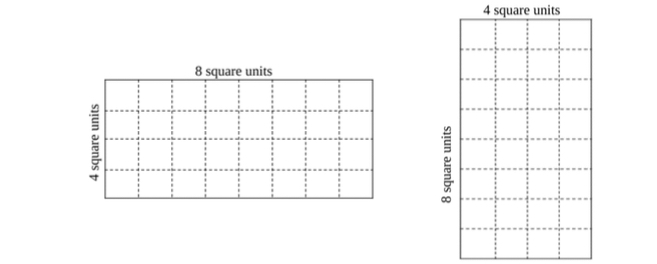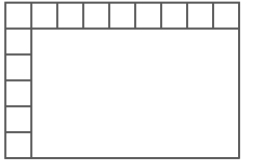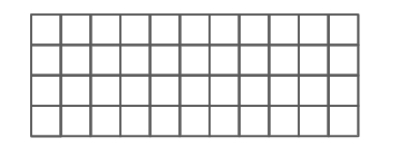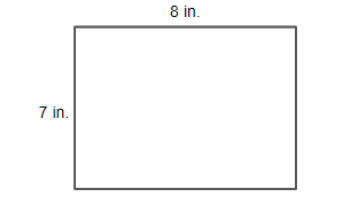# Standard #: MA.3.GR.2.2

This document was generated on CPALMS - www.cpalms.org

Find the area of a rectangle with whole-number side lengths using a visual model and a multiplication formula.

### Clarifications

Clarification 1: Instruction includes covering the figure with unit squares, a rectangular array or applying a formula.

Clarification 2: Two-dimensional figures cannot exceed 12 units by 12 units and responses include the appropriate units in word form.

### General Information

Subject Area: Mathematics (B.E.S.T.)
Strand: Geometric Reasoning
Status: State Board Approved

### Terms from the K-12 Glossary

• Rectangular Array

### Vertical Alignment

Previous Benchmarks

Next Benchmarks

### Purpose and Instructional Strategies

The purpose of this benchmark is for students to calculate the area of rectangles presented visually as arrays or by using a multiplication formula (MTR.5.1).
• The benchmark MA.3.GR.2.1 expects students to calculate the area of rectangles by counting unit squares that covered them with no gaps or overlaps. As students count, they will likely connect their calculations to rectangular arrays and connect understanding that multiplication is a more efficient strategy for calculating than counting or adding unit squares.
• Instruction should encourage students to discover a multiplication formula based on patterns they have observed through practice and classroom discussions. This will make a multiplication formula more meaningful for students conceptually (MTR.5.1). Teachers can help students formalize the formula into an equation, like $A$ = $l$ × $w$. In this benchmark, memorization of a multiplication formula is the goal (MTR.3.1).

### Common Misconceptions or Errors

• When using a formula, students may be confused about which dimension to label the length and width in a rectangle. During instruction, teachers should make connections to the commutative property of multiplication to emphasize that the order in which dimensions are multiplied will not change the rectangle’s area, and therefore the length and width can be labeled flexibly.

### Strategies to Support Tiered Instruction

• Instruction includes the teacher modeling how to draw in rows and columns to cover a figure based on the side lengths given. Students then count the total number of square units that make up the figure and write a multiplication equation to represent it. Teachers help students make the connection to the Commutative Property of Multiplication by having them create and compare figures with the same factors for their rows and columns, just switched. Emphasize that the order in which dimensions are multiplied will not change the rectangle’s area, and therefore the length and width can be labeled flexibly.
• For example, when provided with a figure with the dimensions of 4 × 8, students draw in the rows and columns as shown by the dotted lines. The teacher then asks students to do the same for an image with the dimensions 8 × 4 and has them compare the area of the two figures.• Teacher provides dimensions for a given rectangle and students use square tiles to build the figure in two ways. Students then count the number of tiles in each row and in each column and creates a multiplication expression. Next, the students count the total number of tiles used to make the figure and recognize that as the area of the figure.
• For example, the teacher asks students to create a rectangle with a length of 5 and a width of 7. Students use the square tiles to create two rectangles applying the Commutative Property of Multiplication and writing multiplication equations to match. Then, students count the total number of tiles to check that the area they found for their equation is correct.

Kendra used unit squares with 1-centimeter side lengths to find the area of the rectangle below. She started, but then stopped for a lunch break.• Part A. Write two equations that can be used to find the area of Kendra’s rectangle.
• Part B. What is the area of Kendra’s rectangle?
• Part C. Which has greater area, the rectangle above or a square with side lengths of 8 centimeters? Explain.

### Instructional Items

Instructional Item 1

The rectangle below is composed of unit squares. Which equations can be used to find the area of the rectangle?• a. $A$ = 4 × 10
• b. $A$= 10 × 4
• c. $A$ = 4 + 11
• d. $A$ = 4 × 11
• e. $A$ = 11 + 11 + 11 + 11
• f. $A$ = 11 × 4

Instructional Item 2

What is the area of the rectangle below?*The strategies, tasks and items included in the B1G-M are examples and should not be considered comprehensive.

#### Related Courses

 Course Number1111 Course Title222 5012050: Grade Three Mathematics (Specifically in versions: 2014 - 2015, 2015 - 2022, 2022 and beyond (current)) 7712040: Access Mathematics Grade 3 (Specifically in versions: 2014 - 2015, 2015 - 2018, 2018 - 2022, 2022 and beyond (current)) 5012055: Grade 3 Accelerated Mathematics (Specifically in versions: 2019 - 2022, 2022 and beyond (current)) 5012015: Foundational Skills in Mathematics 3-5 (Specifically in versions: 2019 - 2022, 2022 and beyond (current))

#### Related Access Points

 Access Point Number Access Point Title MA.3.GR.2.AP.2 Find the area of a rectangle with whole-number side lengths by counting unit squares. Explore that the area is the same as what would be found by multiplying the side lengths.

#### Formative Assessments

 Name Description Using Arrays to Model the Distributive Property Students are presented with a rectangular area model and asked to write an equation that represents the distributive property. Cover Me Students determine the area of a rectangle in two different ways.

#### Lesson Plans

 Name Description Best Vegetable Garden The students will plan a vegetable garden, deciding which kinds of vegetables to plant, how many plants of each kind will fit, and where each plant will be planted in a fixed-area garden design. Then they will revise their design based on new garden dimensions and additional plant options.  Students will explore the concept of area to plan their garden and they will practice solving 1 and 2-step real-world problems using the four operations to develop their ideas.Model Eliciting Activities, MEAs, are open-ended, interdisciplinary problem-solving activities that are meant to reveal students’ thinking about the concepts embedded in realistic situations. Click here to learn more about MEAs and how they can transform your classroom. Rectangles Rule! Team Challenge (Lesson 2 of 2) This guided inquiry lesson is designed to follow another CPALMS published lesson #63297 Rectangles Rule! In that first lesson, students used a ruler to measure the side lengths of rectangles and used 1-inch square tiles to find the area of the rectangle. In this lesson, students will use the inquiry process to problem-solve a team challenge: Partition a rectangle into same-size squares using a ruler and determine a formula for finding area (area = length x width). Students will brainstorm, investigate, and share their solutions on how their team worked together to find a solution to the challenge. Same Perimeter, Different Area In this lesson, students are presented with a problem that requires them to create rectangles with the same perimeter but different areas.  Students also search for relationships among the perimeters and areas of different rectangles and find which characteristics produce a rectangle with the greatest area. Bre and Brent the Builders The students will be able to use prior knowledge of the concept of area to relate area to multiplication and discover the formula: base x height. Students will practice by composing two-dimensional squares and rectangles and apply the area formula to build a birdhouse. Area Isn't Just for Squares This lesson helps students make the connections between area and multiplication using square tiles.

#### Original Student Tutorial

 Name Description Terrific Tiling Learn how tilling can be used to find the area of different rectangular rooms in this interactive tutorial.

#### Original Student Tutorial

 Name Description Terrific Tiling: Learn how tilling can be used to find the area of different rectangular rooms in this interactive tutorial.

Printed On:9/28/2023 9:48:16 PM
Print Page | Close this window